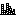#### Vector pixels```#!/usr/bin/env python
# -*- coding: utf-8 -*-

"""  Turns an image into a two-colored grid of vectorpixels: squares,
circles, or squares with rounded corners.

Creating a vector image from a raster image usually consists of
imagining some kind of curve, responsible for the pixels. But this
is guess work at best. Why don’t we work with what’s actually
there—points?

This script turns an image into a grid of vector squares, circles,
or squares with rounded corners.

usage (command line):

python vectorpixel.py inputimage.png > outputimage.svg

TODO: allow multi-colour pictures, implement vertical and horiontal scanlines """

import Image
import sys

class Vectorpixel:
def __init__(self, image):
self.i = Image.open(image).convert("1")
self.constructed = False

def construct(self, grid=24, line=1, rounded=4, test=(lambda x: x == 0)):
self.grid = grid
self.line = line
self.rounded = rounded
self.width = self.height = self.grid - 2 * self.line
self.test = test
self.fill = '#000000'
self.constructed = True

def _yieldlocations(self):
for x in range(self.i.size):
for y in range(self.i.size):
if self.test(self.px[x,y]):
yield (x,y)

def _mkelements(self):
for l in self._yieldlocations():
yield "<rect x='%s' y='%s' width='%s' height='%s' rx='%s' fill='%s'/>" % (
self.grid * l + self.line, self.grid * l + self.line, self.width, self.height, self.rounded, self.fill)

def _format(self):
output = '<svg xmlns="http://www.w3.org/2000/svg" width="%s" height="%s">\n' % (self.i.size * self.grid, self.i.size * self.grid)
for e in self._mkelements():
output += e
output += '\n'
output += '</svg>'
return output

def generate(self):
if not self.constructed:
self.construct()
return self._format()

if __name__ == "__main__":
if len(sys.argv) != 2:
sys.exit("usage (command line):\npython vectorpixel.py inputimage.png > outputimage.svg")
v = Vectorpixel(sys.argv)
v.construct()
print v.generate()```

`sudo easy_install Image`